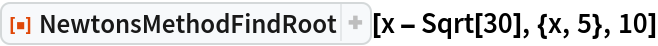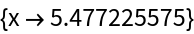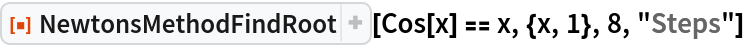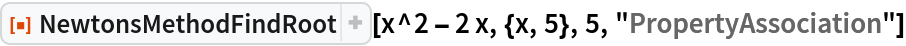Function Repository Resource:

# NewtonsMethodFindRoot

Determine the root of an equation using Newton's method

Contributed by: Jason Martinez
 ResourceFunction["NewtonsMethodFindRoot"][f,{x,x0},tol] searches for a numerical root of f starting at x0 with digits equal to tol. ResourceFunction["NewtonsMethodFindRoot"][lhs⩵rhs,{x,x0},tol] searches for a numerical solution to the equation lhs==rhs. ResourceFunction["NewtonsMethodFindRoot"][f,{x,x0},tol,property] returns a property of the search for the root of f.

## Details

ResourceFunction["NewtonsMethodFindRoot"] supports two options for property:
 "Solution" return root of f "Steps" return a table of steps taken to reach the root
"PropertyAssociation" can be used to return an Association of the properties.

## Examples

### Basic Examples (2)

Find the root of an expression using Newton’s method:

 In:=Out=Determine the steps to find the root of an equation:

 In:=Out=### Scope (1)

Get the property Association of Newton's method:

 In:=Out=## Version History

• 1.0.0 – 15 June 2021